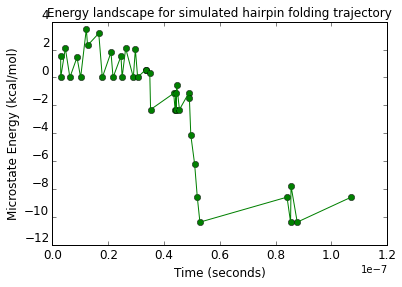# Tutorial 2 - Hairpin trajectories¶

This is a follow-up to Tutorial 1. Here, rather than manually examining secondary structures of interest, we let a simulation run explore the full secondary structure energy landscape according to a Metropolis-biased random walk.

In :
from multistrand.objects import *
from multistrand.options import Options
from multistrand.system import SimSystem, energy


More meaningful names for argument values to the energy() function call, below.

In :
Loop_Energy = 0    # requesting no dG_assoc or dG_volume terms to be added.  So only loop energies remain.
Volume_Energy = 1  # requesting dG_volume but not dG_assoc terms to be added.  No clear interpretation for this.
Complex_Energy = 2 # requesting dG_assoc but not dG_volume terms to be added.  This is the NUPACK complex microstate energy, sans symmetry terms.
Tube_Energy = 3    # requesting both dG_assoc and dG_volume terms to be added.  Summed over complexes, this is the system state energy.


Results of the simulation are stored in the Options object o that was used to set up the simulation. Since this is a "Trajectory Mode" simulation, we will extract the sequence of conformations visited, and print them. Assumes a single strand is being simulated.

In :
def print_trajectory(o):
print o.full_trajectory   # the strand sequence
print o.start_state.structure   # the starting structure
for i in range(len(o.full_trajectory)):
time = o.full_trajectory_times[i]
state = o.full_trajectory[i]
struct = state
dG = state
print struct + ' t=%11.9f seconds, dG=%6.2f kcal/mol' % (time, dG)


Sequence is from Schaeffer's PhD thesis, chapter 7, figure 7.1 -- start with no base pairs formed.

In :
c = Complex( strands=[Strand(name="hairpin", sequence="GTTCGGGCAAAAGCCCGAAC")], structure= 20*'.' )


WARNING! Unfortunately, Multistrand currently does not test to check that the requested structure is valid. Providing an invalid structure is likely to lead to a core dump or segmentation fault.

In :
o = Options(temperature=25, dangles='Some', start_state = [c],
simulation_time = 0.0000001,  # 0.1 microseconds
num_simulations = 1,  # don't play it again, Sam
output_interval = 1,  # record every single step
rate_method = 'Metropolis', # the default is 'Kawasaki' (numerically, these are 1 and 2 respectively)
rate_scaling = 'Calibrated', # this is the same as 'Default'.  'Unitary' gives values 1.0 to both.

In :
print "k_uni = %g /s, k_bi = %g /M/s" % (o.unimolecular_scaling, o.bimolecular_scaling)  # you can also set them to other values if you wantA

k_uni = 4.4e+08 /s, k_bi = 1.26e+06 /M/s


This actually runs the simulation.

In :
s = SimSystem(o)
s.start()

Count: 10 Time: 1.79105536058e-08
Count: 20 Time: 3.36550756928e-08
Count: 30 Time: 4.91143438906e-08


Important caveat: SimSystem will initialize the energy model according to information in Options o if the energy model has not yet been initialized. But if prior calls have already initialized the energy model -- even if it's at another temperature or join_concentration -- then it will not be automatically re-initialized. You would have to do this manually.

In :
print_trajectory(o)

GTTCGGGCAAAAGCCCGAAC
....................
......(......)...... t=0.000000003 seconds, dG=  1.53 kcal/mol
.................... t=0.000000003 seconds, dG=  0.00 kcal/mol
....(........)...... t=0.000000005 seconds, dG=  2.09 kcal/mol
.................... t=0.000000006 seconds, dG=  0.00 kcal/mol
.......(........)... t=0.000000009 seconds, dG=  1.43 kcal/mol
.................... t=0.000000010 seconds, dG=  0.00 kcal/mol
.(........)......... t=0.000000012 seconds, dG=  3.47 kcal/mol
.((......))......... t=0.000000013 seconds, dG=  2.29 kcal/mol
..(......).......... t=0.000000017 seconds, dG=  3.16 kcal/mol
.................... t=0.000000018 seconds, dG=  0.00 kcal/mol
(......)............ t=0.000000021 seconds, dG=  1.79 kcal/mol
.................... t=0.000000022 seconds, dG=  0.00 kcal/mol
......(......)...... t=0.000000025 seconds, dG=  1.53 kcal/mol
.................... t=0.000000025 seconds, dG=  0.00 kcal/mol
....(........)...... t=0.000000026 seconds, dG=  2.09 kcal/mol
.................... t=0.000000029 seconds, dG=  0.00 kcal/mol
....(.........)..... t=0.000000030 seconds, dG=  2.02 kcal/mol
....((.......))..... t=0.000000031 seconds, dG=  0.01 kcal/mol
...(((.......)).)... t=0.000000034 seconds, dG=  0.56 kcal/mol
..((((.......)).)).. t=0.000000034 seconds, dG=  0.57 kcal/mol
.(((((.......)).))). t=0.000000035 seconds, dG=  0.29 kcal/mol
((((((.......)).)))) t=0.000000035 seconds, dG= -2.31 kcal/mol
((((.(.......)..)))) t=0.000000043 seconds, dG= -1.14 kcal/mol
((((((.......)).)))) t=0.000000044 seconds, dG= -2.31 kcal/mol
((((.(.......)..)))) t=0.000000044 seconds, dG= -1.14 kcal/mol
((((((.......)).)))) t=0.000000044 seconds, dG= -2.31 kcal/mol
(((.((.......))..))) t=0.000000045 seconds, dG= -0.54 kcal/mol
((((((.......)).)))) t=0.000000046 seconds, dG= -2.31 kcal/mol
((((.(.......)..)))) t=0.000000049 seconds, dG= -1.14 kcal/mol
((((............)))) t=0.000000049 seconds, dG= -1.45 kcal/mol
(((((..........))))) t=0.000000050 seconds, dG= -4.14 kcal/mol
((((((........)))))) t=0.000000051 seconds, dG= -6.22 kcal/mol
(((((((......))))))) t=0.000000052 seconds, dG= -8.58 kcal/mol
((((((((....)))))))) t=0.000000053 seconds, dG=-10.37 kcal/mol
(((((((......))))))) t=0.000000084 seconds, dG= -8.58 kcal/mol
((((((((....)))))))) t=0.000000085 seconds, dG=-10.37 kcal/mol
.(((((((....))))))). t=0.000000086 seconds, dG= -7.78 kcal/mol
((((((((....)))))))) t=0.000000088 seconds, dG=-10.37 kcal/mol
(((((((......))))))) t=0.000000107 seconds, dG= -8.58 kcal/mol


Note that the simulation proceeds until the time limit has been EXCEEDED. That means that, at the exact time specified, the system is in the PENULTIMATE state.

Just FYI -- but this is important if you are sampling to get an "equilibrium" or time-dependent sample. If you were to take the last state, and if that state is very short-lived, then you would over-sample it.

In :
def myplot():
import numpy as np
import matplotlib
import matplotlib.pylab as plt

times = o.full_trajectory_times
states = o.full_trajectory
energies = [s for s in states]  # you can examine 'states' to see what other useful information is there

plt.figure(1)
plt.plot(times,energies,'go', times,energies,'g-')
plt.title("Energy landscape for simulated hairpin folding trajectory")
plt.xlabel("Time (seconds)",fontsize='larger')
plt.ylabel("Microstate Energy (kcal/mol)",fontsize='larger')
plt.yticks(fontsize='larger',va='bottom')
plt.xticks(fontsize='larger')
plt.show()

In :
%matplotlib inline
myplot()In [ ]: